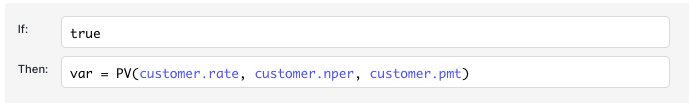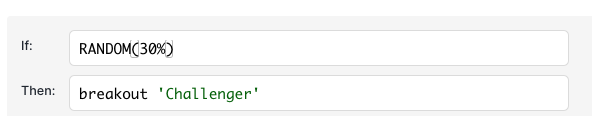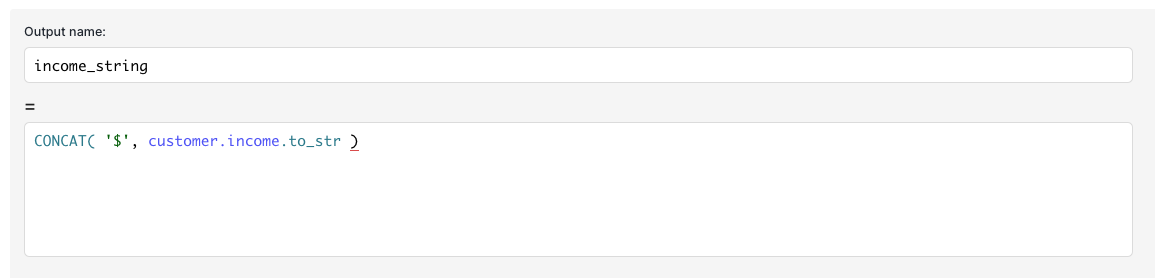# Inline Functions

Functions can be used in:

• Then cells of If/Then Rules
• Action cells of Decision Tables, and Matrix Rules
• Formula rules in Transformation BlocksMost formulas are available under Autocomplete as you type in these cells.

## Inline Functions

PMT - Excel Equivalent

Usage: `PMT(RATE, NPER, PV)`

• Rate - interest rate for loan (expressed in terms of period, so if you're using months, you may have to divide APR by 12)
• Nper - total number of payments for the loan.
• Pv - present value, or the total amount that a series of future payments is worth now; also known as the principal.

PV - Excel Equivalent

Usage: `PV(RATE, NPER, PMT)`

• Rate - interest rate for loan (expressed in terms of period, so if you're using months, you may have to divide APR by 12)
• Nper - total number of payments for the loan.
• Pmt - payment made each period and cannot change over the life of the annuity. Typically has reverse sign of PV to reflect cash inflows/outflows

NPER - Excel Equivalent

Usage: `NPER(RATE, PMT, PV)`

• Rate - interest rate for loan (expressed in terms of period, so if you're using months, you may have to divide APR by 12)
• Pmt - payment made each period and cannot change over the life of the annuity. Typically has reverse sign of PV to reflect cash inflows/outflows
• Pv - present value, or the total amount that a series of future payments is worth now; also known as the principal.

RATE - Excel Equivalent

Usage: `RATE(NPER, PMT, PV)`

• Nper - total number of payments for the loan.
• Pmt - payment made each period and cannot change over the life of the annuity. Typically has reverse sign of PV to reflect cash inflows/outflows
• Pv - present value, or the total amount that a series of future payments is worth now; also known as the principal.

AGE

Usage: `AGE(customer.date_of_birth)`

• input date string in format '2020-05-03'
• returns age in years

AGE_MONTH

Usage: `AGE_MONTH(customer.date_of_birth)`

• input date string in format '2020-05-03'
• returns age in months

AGE_DAY

Usage: `AGE_DAY(customer.date_of_birth)`

• input date string in format '2020-05-03'
• returns age in days

MAX

Usage: `MAX(num1, num2)`

• input two numbers
• returns larger of two

MIN

Usage: `MIN(num1, num2)`

• input two numbers
• returns smaller of two

RANDOM

Usage: `RANDOM(percent_true)`

• returns true `percent_true` of the time
• Useful for champion/challenger flowsROUND

Usage: `ROUND(customer.prop)`

• returns the `customer.prop` rounded to nearest whole number

Usage: `ROUND(customer.prop, places, direction)`

• returns the `customer.prop` rounded to the `places` number of decimal places
• direction can be `up`, `down`, or `nearest` (default)

COALESCE

Usage: `coalesce(item1, item2)`

• returns the first non-null item in the list, similar to SQL coalesce command
• example: `income = coalesce( customer.income, 5000)` will return the customer’s income or 5000 if that field isn’t present in a workflow execution

ABS

Usage: `ABS(transaction.amount)`

• returns the absolute value of a number

SQRT

Usage: `SQRT(transaction.amount)`

• returns the square root of a number

LOG

Usage: `LOG(transaction.number_field)`

• returns the natural logarithm of the number passed into the function

EXP

Usage: `EXP(transaction.power)`

• returns e raised to the power of the number passed into the function

EXPM1

Usage: `EXPM1(transaction.power)`

• returns e^n - 1, where n is the number passed into the function

DISTANCE

Usage: `Distance(pointA.lat, pointA.lon, pointB.lat, pointB.lon)`

• returns distance in meters between two geographic points

## String Functions

CONCAT

Usage: `CONCAT(str1, str2)`

• returns a combined string

SUB_STR

Usage: `SUB_STR(string, start, end)`

• returns a substring, from the starting character to ending character
For example `SUB_STR(john, 1, 3,)` would return oh

SEARCH

Usage: `SEARCH(string, exp)`

• returns true/false if the string contains the expression (accepts regular expressions)
For example `SEARCH(customer.email, 'gmail.com'` would return true for gmail addresses

REPLACE

Usage: `REPLACE(string, find, replace)`

• returns a new string, replacing any instances of the 'find' expression with the 'replace' expression
For example `REPLACE(customer.email, 'gmail.com', 'yahoo.com')` would return a guess at a yahoo email address for a gmail user

EXTRACT

Usage: `EXTRACT(string, regexp)`

• returns a new string, extracting based on the regular expression provided
• For example `EXTRACT(customer.email, '[^@]\*')` would return the username before the '@' sign of an email address

length (dot function)

Usage: `customer.email.length`

• returns the length of a string

## List Functions

Usage: `add( list, item)`

• returns a new list including every item in the original list + the new item

IN

usage: `in( list, item)`

• returns true if the item is in the list, otherwise false
• example: `if: in( approved_phone_types, customer.phone_model) == true`

FILTER

usage: `FILTER( list, itr.field == 'value')`

• filters a list of json objects, and returns only the items with a field equal to a specific value
• example: assuming you have a list of transactions, and want to filter by status == blocked `block_list = FILTER( customer.transactions, itr.status == 'blocked')`

MAP

usage: `MAP( list, itr.field)`

• accepts a list of json objects, and returns a list of all the values for a specific field.
• example: assuming you have a list of transactions with mulitiple fields, and want a list of amounts `amount_list = MAP( customer.transactions, itr.amount)`

GET

usage: `GET( object, key)`

• returns the value for the key, in a given object. you can use this with dynamic keys
• example: say you have a `customer` object, with three pet names as fields (dog, cat, and bird), and a custom variable `type_of_pet` in your workflow that makes it clear the pets you're interested in are dogs. You can get the customer's dog name using `dog_name = GET( customer, type_of_pet)`. it's equivalent to `dog_name = customer.dog` but the benefit is that `type_of_pet` here is dynamic

## Dot Functions (run on a single variable)

to_str

Usage: `customer.income.to_str`

• converts a number to a stringto_dollar

Usage: `customer.income.to_dollar`

• converts a number to a dollar-formatted string (e.g. 500 → \$500.00)

## Data Validation Functions

is_present

Usage: `customer.income.is_present`

• returns true if this field is present for the current Workflow execution, or false if this field is missing

### Type Functions

is_text, is_num, is_boolean, is_list

Usage: `customer.name.is_text`

• returns true if this field is of type "text", "number", etc - otherwise returns false